Publicité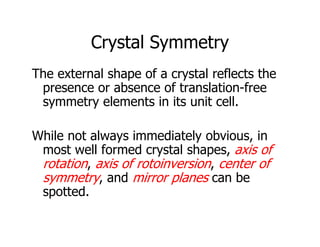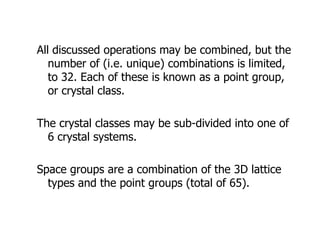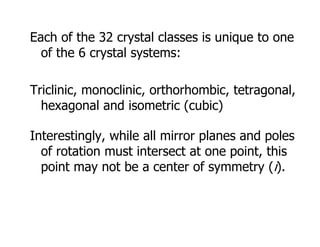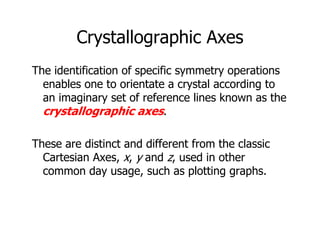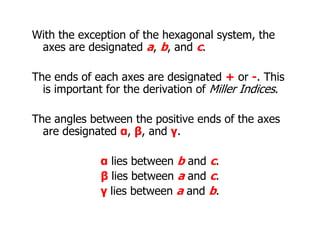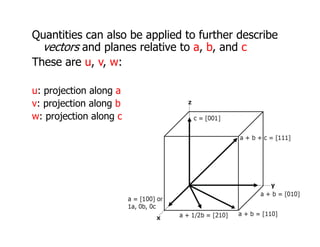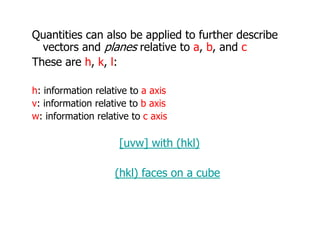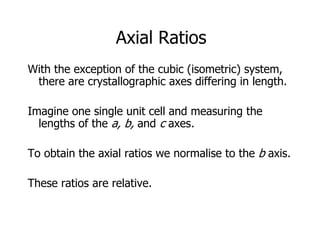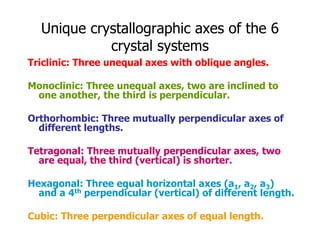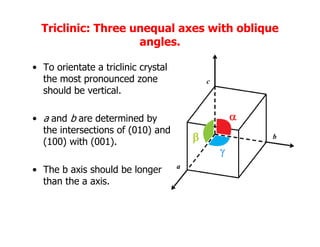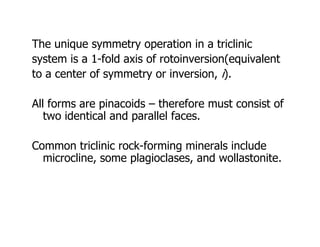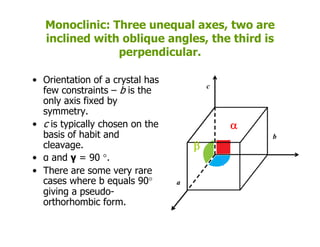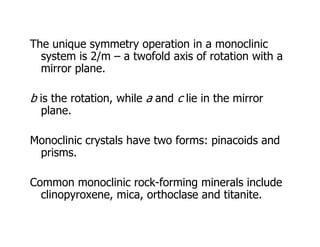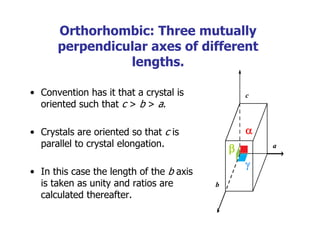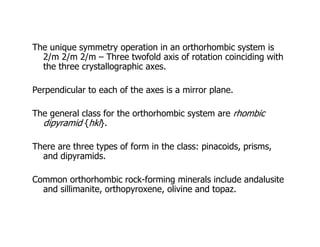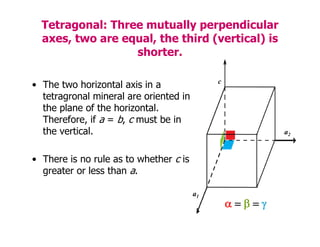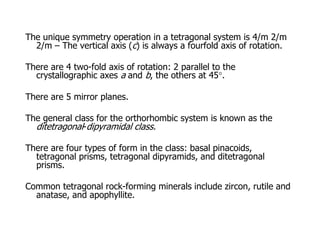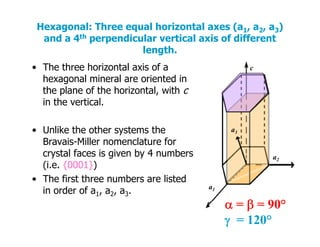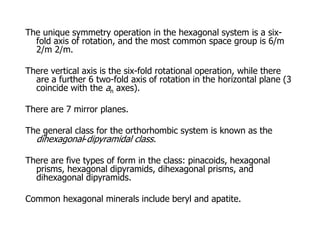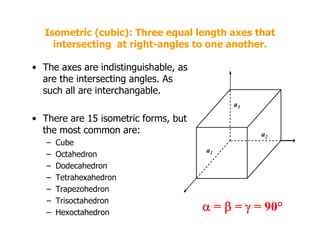1 sur 20
Publicité

### Lecture8.pdf0

1. Crystal Symmetry Crystal Symmetry The external shape of a crystal reflects the The external shape of a crystal reflects the presence or absence of translation-free symmetry elements in its unit cell. y y While not always immediately obvious, in While not always immediately obvious, in most well formed crystal shapes, axis of rotation, axis of rotoinversion, center of , , symmetry, and mirror planes can be spotted.
2. All di d i b bi d b h All discussed operations may be combined, but the number of (i.e. unique) combinations is limited, to 32 Each of these is known as a point group to 32. Each of these is known as a point group, or crystal class. The crystal classes may be sub-divided into one of 6 crystal systems 6 crystal systems. Space groups are a combination of the 3D lattice Space groups are a combination of the 3D lattice types and the point groups (total of 65).
3. Each of the 32 crystal classes is unique to one of the 6 crystal systems: Triclinic, monoclinic, orthorhombic, tetragonal, hexagonal and isometric (cubic) hexagonal and isometric (cubic) Interestingly, while all mirror planes and poles Interestingly, while all mirror planes and poles of rotation must intersect at one point, this point may not be a center of symmetry (i). point may not be a center of symmetry (i).
4. Crystallographic Axes y g p The identification of specific symmetry operations enables one to orientate a crystal according to an imaginary set of reference lines known as the t ll hi crystallographic axes. ff f These are distinct and different from the classic Cartesian Axes, x, y and z, used in other common day usage such as plotting graphs common day usage, such as plotting graphs.
5. With the exception of the hexagonal system, the axes are designated a b and c axes are designated a, b, and c. The ends of each axes are designated + or - This The ends of each axes are designated + or -. This is important for the derivation of Miller Indices. The angles between the positive ends of the axes are designated α, β, and γ. α lies between b and c. β lies between a and c. γ lies between a and b.
6. Quantities can also be applied to further describe vectors and planes relative to a b and c vectors and planes relative to a, b, and c These are u, v, w: u: projection along a v: projection along b p j g w: projection along c
7. Quantities can also be applied to further describe vectors and planes relative to a b and c vectors and planes relative to a, b, and c These are h, k, l: h: information relative to a axis v: information relative to b axis w: information relative to c axis [ ] ith (hkl) [uvw] with (hkl) (hkl) f b (hkl) faces on a cube
8. Axial Ratios With the exception of the cubic (isometric) system, h ll h d ff l h there are crystallographic axes differing in length. I i i l it ll d i th Imagine one single unit cell and measuring the lengths of the a, b, and c axes. To obtain the axial ratios we normalise to the b axis. These ratios are relative.
9. Unique crystallographic axes of the 6 crystal systems crystal systems Triclinic: Three unequal axes with oblique angles. Monoclinic: Three unequal axes, two are inclined to one another, the third is perpendicular. Orthorhombic: Three mutually perpendicular axes of different lengths. Tetragonal: Three mutually perpendicular axes, two are equal, the third (vertical) is shorter. Hexagonal: Three equal horizontal axes (a1, a2, a3) and a 4th perpendicular (vertical) of different length. Cubic: Three perpendicular axes of equal length.
10. Triclinic: Three unequal axes with oblique angles. angles. • To orientate a triclinic crystal the most p ono nced one the most pronounced zone should be vertical. c • a and b are determined by the intersections of (010) and (100) ith (001)   b (100) with (001). • The b axis should be longer   a • The b axis should be longer than the a axis.
11. The unique symmetry operation in a triclinic The unique symmetry operation in a triclinic system is a 1-fold axis of rotoinversion(equivalent to a center of symmetry or inversion, i). to a center of symmetry or inversion, i). All forms are pinacoids – therefore must consist of p two identical and parallel faces. Common triclinic rock-forming minerals include microcline, some plagioclases, and wollastonite.
12. Monoclinic: Three unequal axes, two are inclined with oblique angles, the third is perpendicular. O i t ti f t l h • Orientation of a crystal has few constraints – b is the only axis fixed by t c symmetry. • c is typically chosen on the basis of habit and b  cleavage. • α and γ = 90 °. • There are some very rare   • There are some very rare cases where b equals 90° giving a pseudo- orthorhombic form a orthorhombic form.
13. The unique symmetry operation in a monoclinic The unique symmetry operation in a monoclinic system is 2/m – a twofold axis of rotation with a mirror plane. b is the rotation, while a and c lie in the mirror l plane. Monoclinic crystals have two forms: pinacoids and Monoclinic crystals have two forms: pinacoids and prisms. Common monoclinic rock-forming minerals include clinopyroxene, mica, orthoclase and titanite.
14. Orthorhombic: Three mutually perpendicular axes of different perpendicular axes of different lengths. • Convention has it that a crystal is oriented such that c > b > a. c • Crystals are oriented so that c is  parallel to crystal elongation. • In this case the length of the b axis a   • In this case the length of the b axis is taken as unity and ratios are calculated thereafter. b
15. The unique symmetry operation in an orthorhombic system is The unique symmetry operation in an orthorhombic system is 2/m 2/m 2/m – Three twofold axis of rotation coinciding with the three crystallographic axes. Perpendicular to each of the axes is a mirror plane. The general class for the orthorhombic system are rhombic dipyramid {hkl}. There are three types of form in the class: pinacoids, prisms, and dipyramids. Common orthorhombic rock-forming minerals include andalusite and sillimanite, orthopyroxene, olivine and topaz.
16. Tetragonal: Three mutually perpendicular axes, two are equal, the third (vertical) is shorter. • The two horizontal axis in a tetragronal mineral are oriented in the plane of the horizontal c the plane of the horizontal. Therefore, if a = b, c must be in the vertical. a2 • There is no rule as to whether c is greater or less than a greater or less than a. a1      
17. The unique symmetry operation in a tetragonal system is 4/m 2/m 2/m – The vertical axis (c) is always a fourfold axis of rotation 2/m – The vertical axis (c) is always a fourfold axis of rotation. There are 4 two-fold axis of rotation: 2 parallel to the crystallographic axes a and b the others at 45° crystallographic axes a and b, the others at 45 . There are 5 mirror planes. The general class for the orthorhombic system is known as the ditetragonal-dipyramidal class. There are four types of form in the class: basal pinacoids, tetragonal prisms, tetragonal dipyramids, and ditetragonal prisms. Common tetragonal rock-forming minerals include zircon, rutile and anatase, and apophyllite.
18. Hexagonal: Three equal horizontal axes (a1, a2, a3) and a 4th perpendicular vertical axis of different length. • The three horizontal axis of a he agonal mine al a e o iented in c hexagonal mineral are oriented in the plane of the horizontal, with c in the vertical. • Unlike the other systems the B i Mill l t f a3 Bravais-Miller nomenclature for crystal faces is given by 4 numbers (i.e. {0001}) a2 • The first three numbers are listed in order of a1, a2, a3. a1  90°  =  = 90°  = 120°
19. The unique symmetry operation in the hexagonal system is a six- fold axis of rotation, and the most common space group is 6/m , p g p 2/m 2/m. There vertical axis is the six-fold rotational operation, while there f th 6 t f ld i f t ti i th h i t l l (3 are a further 6 two-fold axis of rotation in the horizontal plane (3 coincide with the an axes). There are 7 mirror planes There are 7 mirror planes. The general class for the orthorhombic system is known as the dihexagonal-dipyramidal class dihexagonal-dipyramidal class. There are five types of form in the class: pinacoids, hexagonal prisms hexagonal dipyramids dihexagonal prisms and prisms, hexagonal dipyramids, dihexagonal prisms, and dihexagonal dipyramids. Common hexagonal minerals include beryl and apatite. Common hexagonal minerals include beryl and apatite.
20. Isometric (cubic): Three equal length axes that intersecting at right-angles to one another intersecting at right angles to one another. • The axes are indistinguishable, as a e the inte secting angles As are the intersecting angles. As such all are interchangable. a3 • There are 15 isometric forms, but the most common are: a2 3 – Cube – Octahedron – Dodecahedron a1 – Tetrahexahedron – Trapezohedron – Trisoctahedron Trisoctahedron – Hexoctahedron  =  =  = 90°
Publicité